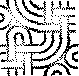Please feel free to experiment here, after the four dashes below... and please do NOT create new pages without any meaningful content just to try it out!

Tip: Shift-click "HelpOnEditing" to open a second window with the help pages.

## Formatting

italic bold typewriter

backtick typewriter (configurable)

bigger smaller

preformatted some more
and some more lines too

   1 def syntax(highlight):
2     print "on"
3     return None## SmileysAlert

## Lists

### Bullet

• first
1. nested and numbered
2. numbered lists are renumbered
• second
• third
• mal sehen
blockquote
• deeper

Term
Definition

# IRC Log test

   1 (23:18) <     jroes> ah
2 (23:19) <     jroes> hm, i like the way {{{ works, but i was hoping the lines would wrap
3 (23:21) -!- gpciceri [~gpciceri@host181-130.pool8248.interbusiness.it] has quit [Read error: 110 (Connection timed out)]
4 (23:36) < ThomasWal> you could also write a parser or processor
5 (23:38) <     jroes> i could?
6 (23:38) <     jroes> would that require modification on the moin end though?
7 (23:38) <     jroes> i cant change the wiki myself :x
8 (23:39) < ThomasWal> parsers and processors are plugable
9 (23:39) < ThomasWal> so you dont need to change the core code
10 (23:40) < ThomasWal> you need to copy it to the wiki data directory though
11 (23:40) <     jroes> well, what i meant to say was that i dont have access to the box running the wiki
13 (23:40) <     jroes> yeah :/


digraph G {
node [fillcolor=white, style=filled];
0 [label="And"];
0 -> 1;
1 [label="a", shape=box];
0 -> 2;
2 [label="Or"];
2 -> 1;
2 -> 3;
3 [label="b", shape=box];
0 -> 4;
4 [label="Or"];
4 -> 3;
4 -> 5;
5 [label="c", shape=box];

standard formula: If f is entire (holomorphic on latex2(\usepackage{dsfont} % $$\mathds{C}$$)) and without zeroes, for every closed curve latex2($$\gamma$$) the integral latex2($$\oint_\gamma \frac{f'(z)}{f(z)}dz$$) is zero.

This is a red square:

\usepackage{graphics,color}
%%end-prologue%%
\newsavebox{\mysquare}
\savebox{\mysquare}{\textcolor{red}{\rule{1in}{1in} } }
\usebox{\mysquare}

This is a Z notation:

\usepackage{oz}
\setlength{\zedcornerheight}{0.2em}
%%end-prologue%%
\small
\begin{schema}{level2\_robot}
\textbf{in~} x: \mathbb{B} \\
\textbf{in~} feeling: \mathbb{R} \\
\textbf{in~} control: \mathbb{C} \\
\zbreak
\textbf{out~} moving: \mathbb{R} \\
\textbf{out~} u: \mathbb{B} \\
\ST
\zbreak
control.1.1 = x2moving \imp \\
\t1 maxrate(x, $10K$) \\
\t1 \land delay(pr''(x \copyright DBH), moving, \Delta_{level2\_robot}) \\
\zbreak
control.1.1 = feeling2u \imp \\
\t1 maxrate(u, $10K$) \\
\t1 \land delay(feeling, pr''(u \copyright IBH), \Delta_{level2\_robot}) \\
\zbreak
control.1.1 = x2moving\_feeling2u \imp \\
\t1 maxrate(x, $10K$) \\
\t1 \land maxrate(u, $10K$) \\
\t1 \land delay(pr''(x \copyright DBH), moving, \Delta_{level2\_robot}) \\
\t1 \land delay(feeling, pr''(u \copyright IBH), \Delta_{level2\_robot}) \\
\end{schema}

JunHu: WikiSandBox (last edited 2013-12-27 21:52:00 by JunHu)